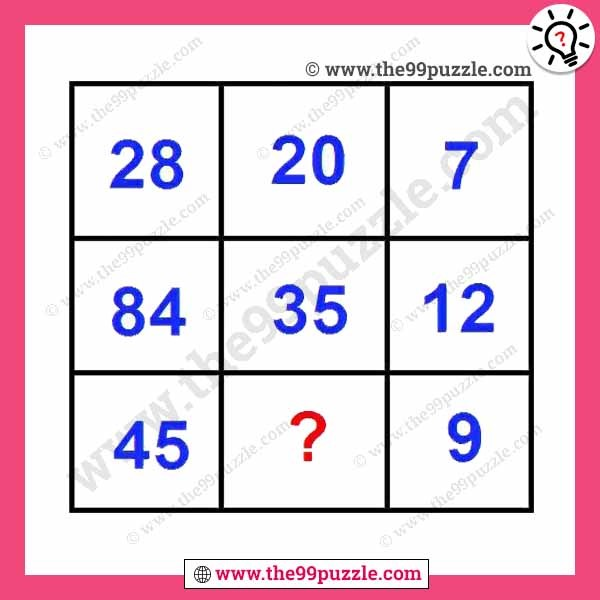# Can you replace the question mark in this box puzzle? – Puzz137

Can you replace the question mark in this box puzzle? These math puzzles are interesting and challenging. A logical reasoning math problem can improve your problem-solving skills. If you are a genius replace the question mark in this box puzzle.###### Explanation:

1st row=28÷7×5=20

2nd row=84÷12×5=35

3rd row=45÷9×5=25